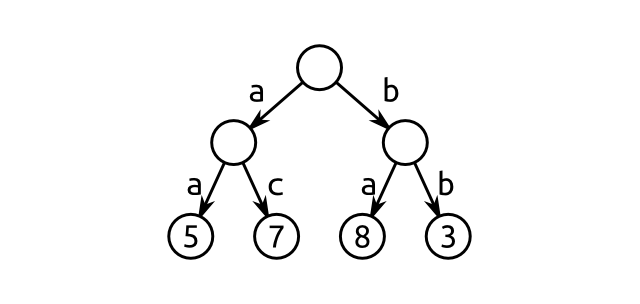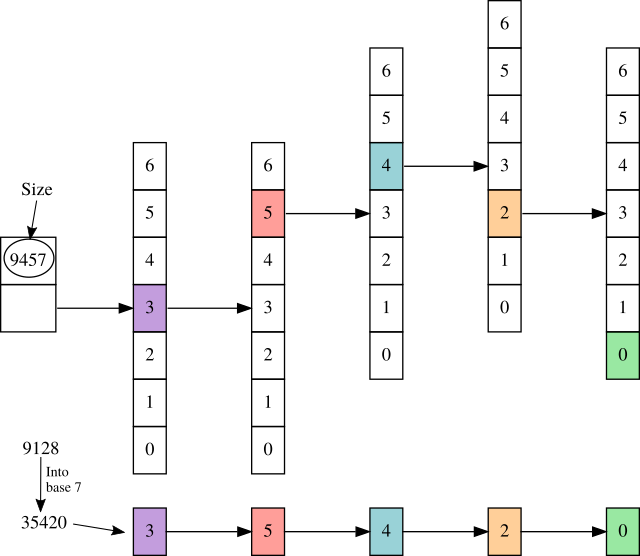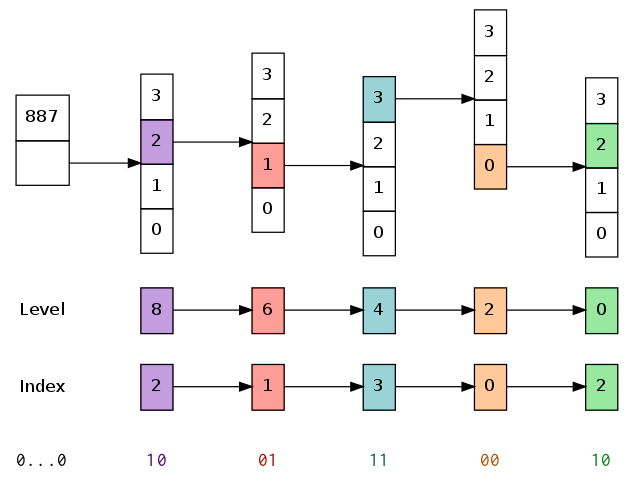# Understanding Clojure's Persistent Vectors, pt. 2

posted

In the previous post about Clojure’s persistent vectors (read it, if you haven’t already), we kind-of understood how insertion, updates and popping elements in a vector worked. We did not really understand how to get to the right element, and I will cover that along with how lookup works in this post.

To understand how we pick the right branch, I think it’s good to give the proper name of the structure and explain why it is named that. It sounds a bit weird to explain branching through the name of a data structure, but it makes sense when you consider that such a name may describe how it works.

## Naming

A more formal name for Clojure’s persistent vector structure is persistent bit-partitioned vector trie1. What I explained in the previous post was how persistent digit-partitioned vector tries work. Don’t worry, a bit-partitioned one is just an optimized digit-partitioned trie, and in the previous post there is nothing different about them. In this one, there is a small difference related to performance. It has otherwise no practical differences.

I guess many of you don’t know all of those words I mentioned in the above paragraph, so let’s describe them, one by one.

## Persistence

In the last post, I used the word persistent. I said we want to be “persistent”, but didn’t really explain what persistence itself really means.

A persistent data structure doesn’t modify itself: Strictly speaking they, don’t have to be immutable internally, just have to be perceived as such. Whenever you do “updates”, “inserts” and “removals” on a persistent data structure, you get a new data structure back. The old version will always be consistent, and whenever given some input in, it will always spit out the same output.

When we talk about a fully persistent data structure, all versions of a structure should be updateable, meaning that all possible operations you can do on a version can be performed on another. In early “functional data structure” time, it was common to “cheat” with the structures and make the older versions “decay” over time by mutating the internals, making them slower and slower compared to the newer versions. However, Rich Hickey decided that all the versions of Clojure’s persistent structures should have the same performance guarantees, regardless of which version of the structure you are using.

## Vector

A vector is a one-dimensional growable array. C++’s `std::vector` and Java’s `java.util.ArrayList` are examples of mutable implementations. There’s not much more to it than that, really. A vector trie is a trie which represents a vector. It doesn’t have to be persistent, but in our case, it is.

## Trie

Tries are a specific type of trees, and I think it’s best to show the actual difference by explaining the more known trees first.

In RB-trees and most other binary trees, mappings or elements are contained in the interior nodes. Picking the right branch is done by comparing the element/key at the current node: If the element is lower than the node element, we branch left, and if it is higher, we branch right. Leaves are usually null pointers/nil, and doesn’t contain anything.

The RB-tree above is taken from Wikipedia’s article on RB-trees. I’m not going to explain how those work in detail, but let us take a tiny example on how we check if 22 is contained in the RB-tree:

1. We start at the root, 13, and compare it with 22. As 13 < 22, we go right.
2. The new node has 17 in it, and compare it with 22. As 17 < 22, we still go right.
3. The next node we’ve walked into is 25. As 25 > 22, we go left.
4. The next node is 22, so we know that 22 is contained in the tree.

If you want a good explanation of how RB-trees work, I would recommend Julienne Walker’s Red Black Tree Tutorial.

A trie, on the other hand, has all the values stored in its leaves2. Picking the right branch is done by using parts of the key as a lookup. Consequently, a trie may have more than two branches. In our case, we may have as many as 32!An example of a general trie is illustrated in the figure above. That specific trie is a map: It takes a string of length two and returns an integer represented by that string if it exists in the trie. `ac` has the value 7, whereas `ba` has the value 8. Here’s how the trie works:

For strings, we split the string into characters. We then take the first character, find the edge represented by this value, and walk down that edge. If there is no edge for that value, we stop, as it is not contained in the trie. If not, we continue with the second character, and so on. Finally, when we are done, we return the value if it exists.

As an example, consider `ac`. We do as follows:

1. We split `ac` up into `[a, c]`, and start at the root node.
2. We check if there is an edge in the node for `a`, and there is: We follow it.
3. We check if there is an edge in the node for `c`, and there is. We follow that as well.
4. We have no more characters left, which means the current node contains our value, 7. Therefore, we return 7.

Clojure’s Persistent Vector is a trie where the indices of elements are used as keys. But, as you may guess, we must split up the index integers in some way. To split up integers, we either use digit partitioning or its faster sibling, bit partitioning.

## Digit Partitioning

Digit partitioning means that we split up the key into digits, which we then use as a basis for populating a trie. For instance, we can split up the key `9128` to `[9, 1, 2, 8]`, and put an element into a trie based on that. We may have to pad with zeroes at the front of the list if the depth of the trie is larger than the size of the list.

We can also use whatever base we would like, not just base 10. We would then have to convert the key to the base we wanted to use, and use the digits from the conversion. As an example, consider `9128` yet again. `9128` is `35420` in base 7, so we would have to use the list `[3, 5, 4, 2, 0]` for lookup/insertion in the trie.The trie (laying sideways, without the edges and nodes we’re not walking) above shows how we traverse a digit-partitioned trie: We pick the most significant digit, in this case, 3, and walk that specific branch. We continue with the second most significant digit in the same manner, until we have no digits left. When we’ve walked the last branch, the object we’re standing with — the first object in the rightmost array in this case — is the object we wanted to look up.

Implementing such a lookup scheme is not too hard if you know how to find the digits. Here’s a Java version where everything not related to lookup is stripped away:

``````public class DigitTrie {
public static final int RADIX = 7;

// Array of objects. Can itself contain an array of objects.
Object[] root;
// The maximal size/length of a child node (1 if leaf node)
int rDepth; // equivalent to RADIX ** (depth - 1)

public Object lookup(int key) {
Object[] node = this.root;

// perform branching on internal nodes here
for (int size = this.rDepth; size > 1; size /= RADIX) {
node = (Object[]) node[(key / size) % RADIX];
// If node may not exist, check if it is null here
}

// Last element is the value we want to lookup, return it.
}
}
``````

The `rDepth` value represents the maximal size of a child of the root node: A number with n digits will have `RADIX` to the power of n possible values, and we must be able to put them all in the trie without having collisions.

In the for loop within the `lookup` method, the value `size` represents the maximal size a child of the current node can have. For each child we go over, that size is decremented by the branching factor, i.e. the radix or base of the digit trie.

The reason we’re performing a modulo operation on the result is to ignore the more significant digits — digits we’ve branched on earlier. We could potentially remove the higher digit from the key every time we branch into a child, but the code would be a tiny bit more complicated in that case3.

## Bit Partitioning

Digit-partitioned tries would generally have to do a couple of integer divisions and modulo operations. Doing this is on every branch we must take is a bit time-consuming. We would, therefore, like to speed this part up if it is possible.

So, as you may guess, bit-partitioned tries are a subset of the digit-partitioned tries. All digit-partitioned tries in a base which is a power of two (2, 4, 8, 16, 32, etc) can be turned into bit-partitioned ones. With some knowledge of bit manipulation, we can remove those costly arithmetic operations.

Conceptually, it works in the same way as digit partitioning does. However, instead of splitting the key into digits, we split it into chunks of bits with some predefined size. For 32-way branching tries, we need 5 bits in each part, and for 4-way branching tries, we need 2. In general, we need as many bits as the size of our exponent.

So, why is this faster? By using bit tricks, we can get rid of both integer division and modulo. If `power` is two to the power of `n`, we can use that ```x / power == x >>> n```4 and `x % power == x & (power - 1)`. These formulas are just identities related to how integers are represented internally, namely as sequences of bits.

If we use this result and combine it with the previous implementation, we end up with the following code:

``````public class BitTrie {
public static final int BITS = 5,
WIDTH = 1 << BITS, // 2^5 = 32
MASK = WIDTH - 1; // 31, or 0x1f

// Array of objects. Can itself contain an array of objects.
Object[] root;
// BITS times (the depth of this trie minus one).
int shift;

public Object lookup(int key) {
Object[] node = this.root;

// perform branching on internal nodes here
for (int level = this.shift; level > 0; level -= BITS) {
node = (Object[]) node[(key >>> level) & MASK];
// If node may not exist, check if it is null here
}

// Last element is the value we want to lookup, return it.
}
}
``````

This is more or less exactly what Clojure’s implementation is doing! See these lines of the Clojure code to verify it; The only difference is that it performs boundary checks and a tail check as well.

The important thing to note here is that we’ve not only changed the operators, but we’ve also replaced the `rDepth` value with a `shift` value. Instead of storing the whole value, we’re only storing the exponent. This makes us able to use bit shifting on the key, which we use in the `(key >>> level)` part. The other parts should be fairly straightforward to understand, given that one knows bit operations well. However, let’s take an example for the ones unfamiliar with such tricks. The explanation is quite thorough, so feel to skip parts you understand.

Say we only have 2-bit partitioning (4-way branching) instead of 5 bits (32-way) for visualization purposes. If we want to look up a value in a trie with 887 elements, we would have a `shift` equal to 8: All the children of the root node can contain at most `1 << 8 == 256` elements each. The width and mask are also changed by the bit count: The mask will here be 3 instead of 31.Say we want to look up the contents of the element with key 626. 626 in its binary representation is `0000 0010 0111 0010`. Following the algorithm, step by step, both written above and within Clojure’s source code, we would have to do the following:

• `node` is set up to be the root note, and `level` is set up to be `8`.
• As `level` is over 0, we start the for loop.
• We perform the operation `key >>> level` first. In this case, this is `626 >>> 8`, which cuts away the first 8 bits: We’re left with `0000 0010` or 2 in decimal.
• We perform the masking: `(key >>> level) & MASK == 2 & 3`. The masking sets all the bits except the first two to zero. This yields no difference here: We still have 2.
• We replace the current `node` with its child at index 2.
• We decrement `level` by 2, and set it to `6`.
• As `level` is over 0, we continue the for loop.
• We again perform the operation `key >>> level == 626 >>> 6`. This cuts away the last 6 bits, and we’re left with `0000 0010 01`, or 9 in decimal.
• We perform the masking: `(key >>> level) & MASK == 9 & 3`, or `1001 & 0011` in binary. This shaves off the top `10`, and we’re left with `01`, or 1 in decimal.
• We replace the current `node` with its child at index 1.
• We decrement `level` by 2, and set it to `4`.
• As `level` is over 0, we continue the for loop
• Same trick here again. `626 >>> 4` leaves us with the bits `0010 0111`.
• The mask sets all but the 2 first bits to zero, and we’re left with `11`.
• We replace the current `node` with its child at index `0b11 == 3`.
• We decrement `level` by 2, and set it to `2`.
• As `level` is over 0, we continue the for loop
• `626 >>> 2` leaves us with `0010 0111 00`.
• The 2 first bits are `0b00 == 0`.
• We replace `node` with its first child, the one at index 0.
• We decrement `level` by 2, and set it to `0`.
• As `level` is (finally) not over 0, we jump over the for loop.
• We mask `key` with the mask, and get back the bits `10`. We return the contents at index 2 from `node`.

That is almost every single machine instruction you would have to perform to lookup a value in a Clojure vector, with a depth of 5. Such a vector would contain between 1 and 33 million elements. The fact that the shifts and masks are some of the most efficient operations on a modern CPU makes the whole deal even better. From a performance perspective, the only “pain point” left on lookups are the cache misses. For Clojure, that is handled pretty well by the JVM itself.

And that’s how you do lookups in tries and in Clojure’s vector implementation. I would guess the bit operations are the hardest one to grok, everything else is actually very straightforward. You just need a rough understanding of how tries work, and that’s it!

If you’ve finished this, then part 3 is next up, explaining how the tail, an optimisation of the vector, works.

1. You could probably discuss whether this is the formal name if you want to be pendantic. Daniel Spiewak calls them “Bitmapped Vector Tries” in his talk “Extreme Cleverness”. In the Clojure community, they tend to either get under the name vector or persistent vector. The goal is not to provide the name it is most known under, but a name explaining the most important features of the data structure for this blog series: Namely that it is a persistent trie emulating a vector by bit-partitioning the indices of the elements.

2. I’m lying a bit here, to make the explanation easier: Some trie variants may actually have values stored in internal nodes, but the ones we will work with will not.

3. However, it may be faster! The `div` operator in x86 would store the quotient and remainder in two different registers, so we can piggyback the division we have to do anyway. The only remaining work is thus a `subl`, which will be faster than a modulo operation. If you’re in need of implementing a very fast digit-partitioned trie which is not a power of two, take note.

But this depends on the specific radix you are working with. In certain cases, a division can be performed through a series of shifts and multiplications, and a modulo can be optimized the same way.

4. A small note here: In Java, `>>>` is a logical shift (any bits shifted in will be zero), and `>>` is an arithmetic shift (bits shifted in will have the same value as the most significant bit). There is effectively no difference as long as we don’t use the signed bit, although I would guess `>>>` may be faster than `>>` on some machine architectures.

For C/C++/Go and friends, `>>>` is the same as `>>` for unsigned types.